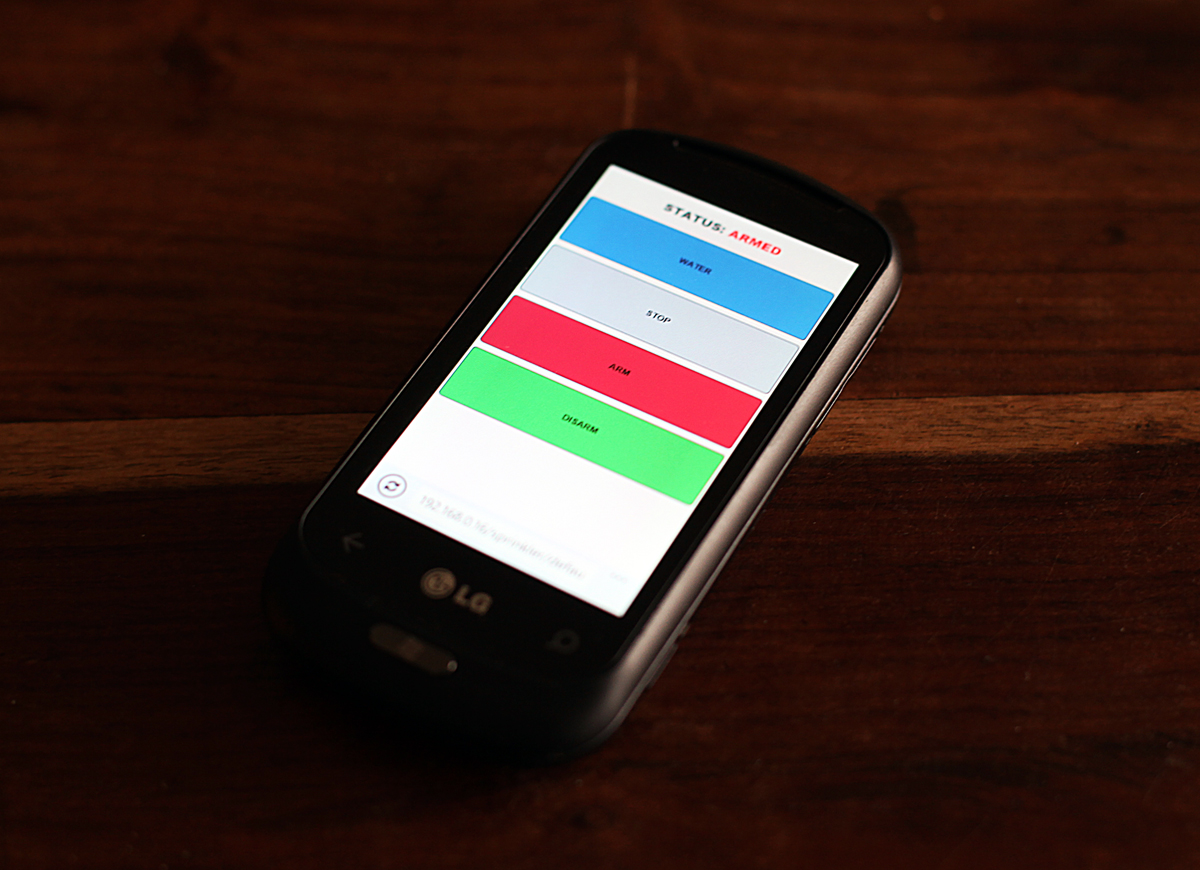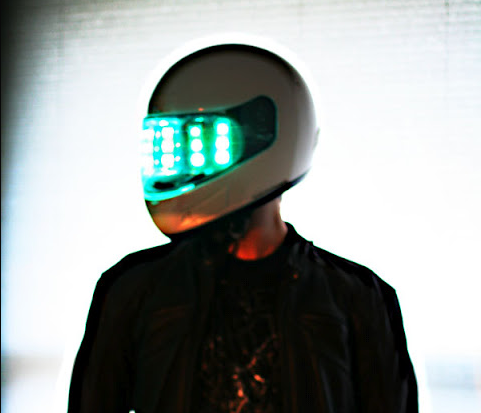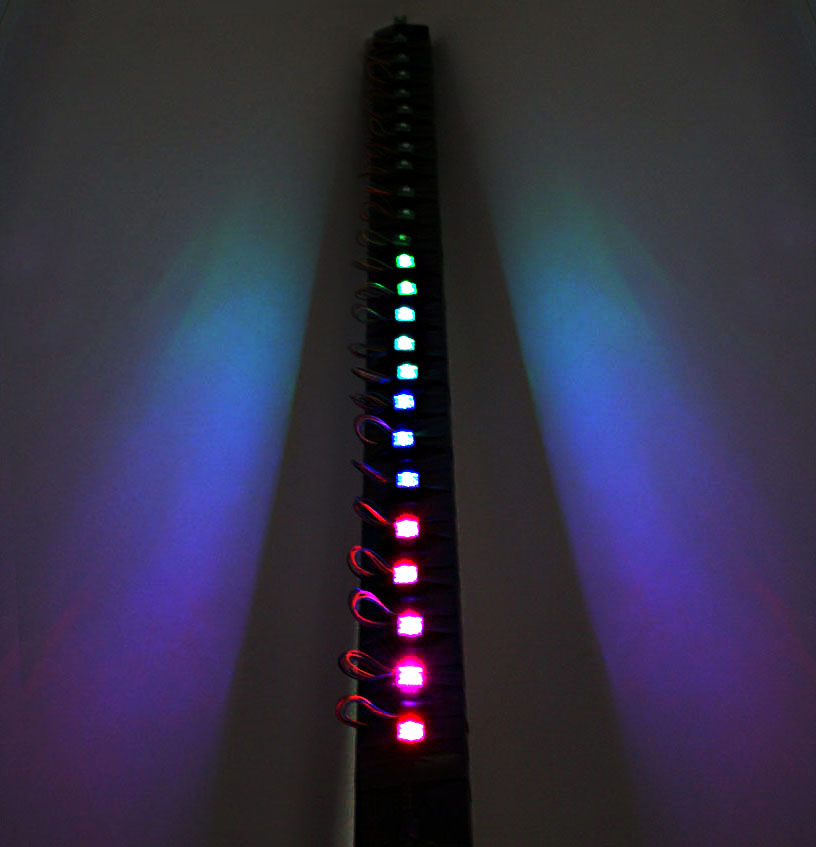# Tag Archives: arduino - Page 2

## “Spaghetti” IP Cam / Arduino Motion Detect SprinklerAfter a neighbourhood dog decided my front lawn was a fantastic place to poop on and his owners decided that they don’t care for bylaws I set upon finding a solution. Sure you could try cayenne pepper, mothballs, ammonia or even marking your own territory (take that!) but I already had an IP camera monitoring my front yard for security purposes, so I figured I’d just hook up an Arduino and a sprinkler valve. This yard defense solution has two added benefits, it keeps my lawn healthy (I can set up timed watering through this system) and it sends offending dogs home stinkin’ wet. From me having to shovel dookie off my lawn to negligent owners having to deal with wet dog — perfect.

When the IP camera detects motion in configured regions of its video stream (the rectangles in the screenshot) it triggers one of its General Purpose Input/Output ports (GPIO). The Arduino is listening for this GPIO signal and once its received the Arduino triggers a relay which connects a 24v power supply to the sprinkler solenoid valve. The valve opens when 24v is applied to it and “sprinkles” whatever was responsible for the motion.

The TRENDnet IP camera I employed works great as it already records video of events to network or attached storage, sends email alerts with snapshots and allows manual triggering of its GPIO.

The TRENDnet monitoring plugin only works in IE but after some reverse engineering and C# coding I had an easy to use web interface for all browsers and mobile devices.

If there’s enough interest I’ll post circuit and wiring diagrams. Ensure the valves you get are non-latching 24v solenoids, some 9v valves seem tempting but are magnetic and require a more complex circuit.

Why Spaghetti? Watch more ATHF.

#### Parts

Update: Some folks have asked why not just trigger the valve relay directly from the GPIO on the camera. This could’ve been done, but then I wouldn’t have gotten as much control as I wanted. This way I can configure timing to prevent the sprinkler itself from setting off the motion detector, set up timed watering as well as trigger other devices at various timings (DSLR for reaction shots). Another addition may be an XBee based remote control or hardwired buttons for various functions.

Update: I’ve added a quick circuit diagram and simple Arduino code.

#### Arduino Code

```
int gpioPin = 1;       			// GPIO input
int val = 0;           			// value read from GPIO input
int trigPin = 13;      			// solenoid relay output

void setup()
{
pinMode(trigPin, OUTPUT);
}

void loop()
{
if (val > 500)
{
// GPIO triggered, open the valve
digitalWrite(trigPin, HIGH);
}
else
{
// GPIO off, close the valve
digitalWrite(trigPin, LOW);
}
}```

## Ghetto Daft Punk HelmetOh the grandest of plans I had, to create an ultra slick Daft Punk Helmet replica (as others have done), alas time ran out so I just crammed one together. A bunch of ShiftBrites (stolen from my Wine-O-Meter) driven by an Ardunio, mounted on some clear plastic and shoved inside one of my motorcycle helmets. Definitely not a high end deal, but it certainly was fun and made for some pretty swag photos.

As a side note, this used horrible sledgehammer style coding as it was a rush job, keep that in mind if you use the sketch below.

#### Arduino Sketch

```#include <HughesyShiftBrite.h>

HughesyShiftBrite sb;
#define NumLEDs 20
#define NumChannels 3
/*
10 = Data
11 = Latch
12 = Enable
13 = Clock
*/
#define SIZE    255
#define DELAY    10
#define HUE_MAX  6.0

float HUE_DELTA = 0.01;

int maxVal = 1023;
int count = 0;
int largecount = 0;
int trailcount = 0;
int animation = 0;

int currentVal = 0;
int currentChannel = 0;
int LEDChannels[NumLEDs][NumChannels] = {0};
int displayValue = 20;

int zigzagdirection = 0;
int zigzagswap = 0;

long deltas = {5, 6, 7 };
long rgb;
long trailhue;
long rgbval;
long k, temp_value;

int r1;
int g1;
int b1;

// for reasons unknown, if value !=0, the LED doesn't light. Hmm ...
// and saturation seems to be inverted
float hue=0.0, saturation=1.0, value=1.0;

void setup()
{
sb = HughesyShiftBrite(10,11,12,13);

for (k=0; k<3; k++) {
rgb[k]=0;
if (random(100) > 50) {
deltas[k] = -1 * deltas[k]; // randomize direction
}
}
}

void loop()
{
count++;
memset(LEDChannels, 0, sizeof(LEDChannels));

nextcolor();

for (int z = 1;z<100;z++)
{
trailhue[z] = trailhue[z-1];
trailhue[z] = trailhue[z-1];
trailhue[z] = trailhue[z-1];
}

trailhue = rgb;
trailhue = rgb;
trailhue = rgb;

if(largecount > 30 && random(20) == 0)
{
r1 = random(80);
g1 = random(80);
b1 = random(80);
animation = random(10);
largecount = 0;

if(animation == 7){
HUE_DELTA = .5;
}else if(animation > 7)
{
HUE_DELTA = 0.5;
}
else{
HUE_DELTA = 0.01;
}
}

if(animation==0)
{
sidescroll(count,rgb,rgb,rgb);

//if(count > 1)
//{
//  sidescroll(count-1,trailhue,trailhue,trailhue);
//}
}
else if(animation==1)
{
downscroll(count,rgb,rgb,rgb);
}
else if(animation==2)
{
zigzag(count,rgb,rgb,rgb);
}
else if(animation==3)
{
}
else if(animation==4)
{
}
else if(animation==5)
{
contrast(count,rgb,rgb,rgb);
}
else if(animation==6)
{
randompattern();
}
else if(animation==7)
{
rainbow(count);
}
else
{
circle(count,rgb,rgb,rgb);
}

for (int h = 1;h<=NumLEDs-2;h++)
sb.sendColour(LEDChannels[h],LEDChannels[h],LEDChannels[h]);

delay(50);
if(count > 5){
count = 0;
largecount++;
}
sb.latch();
}

void randomhue()
{
for (int h = 1;h<=NumLEDs-2;h++)
{
//        LEDChannels
}
}

void nextcolor()
{
hue += HUE_DELTA;
if (hue > HUE_MAX) {
hue=0.0;
}
rgbval = HSV_to_RGB(hue, saturation, value);
rgb = (rgbval & 0x00FF0000) >> 16; // there must be better ways
rgb = (rgbval & 0x0000FF00) >> 8;
rgb = rgbval & 0x000000FF;
}

void rainbow(int count)
{
long oldrgb;
oldrgb = rgb;
oldrgb = rgb;
oldrgb = rgb;

float oldhue = hue;

for (int h = 1;h<=6;h++)
{
LEDChannels[13-h] = rgb;
LEDChannels[13-h] = rgb;
LEDChannels[13-h] = rgb;
LEDChannels[h] = rgb;
LEDChannels[h] = rgb;
LEDChannels[h] = rgb;
LEDChannels[12+h] = rgb;
LEDChannels[12+h] = rgb;
LEDChannels[12+h] = rgb;
nextcolor();
}

rgb = oldrgb;
rgb = oldrgb;
rgb = oldrgb;
hue = oldhue;
}

void contrast(int count, int r, int g, int b)
{

if(count>3)
{
LEDChannels[13-1] = r;
LEDChannels[13-1] = g;
LEDChannels[13-1] = b;
LEDChannels[13-2] = r1;
LEDChannels[13-2] = g1;
LEDChannels[13-2] = b1;
LEDChannels[13-3] = r;
LEDChannels[13-3] = g;
LEDChannels[13-3] = b;
LEDChannels[13-4] = r1;
LEDChannels[13-4] = g1;
LEDChannels[13-4] = b1;
LEDChannels[13-5] = r;
LEDChannels[13-5] = g;
LEDChannels[13-5] = b;
LEDChannels[13-6] = r1;
LEDChannels[13-6] = g1;
LEDChannels[13-6] = b1;

LEDChannels = r;
LEDChannels = g;
LEDChannels = b;
LEDChannels = r1;
LEDChannels = g1;
LEDChannels = b1;
LEDChannels = r;
LEDChannels = g;
LEDChannels = b;
LEDChannels = r1;
LEDChannels = g1;
LEDChannels = b1;
LEDChannels = r;
LEDChannels = g;
LEDChannels = b;
LEDChannels = r1;
LEDChannels = g1;
LEDChannels = b1;

LEDChannels[12+1] = r;
LEDChannels[12+1] = g;
LEDChannels[12+1] = b;
LEDChannels[12+2] = r1;
LEDChannels[12+2] = g1;
LEDChannels[12+2] = b1;
LEDChannels[12+3] = r;
LEDChannels[12+3] = g;
LEDChannels[12+3] = b;
LEDChannels[12+4] = r1;
LEDChannels[12+4] = g1;
LEDChannels[12+4] = b1;
LEDChannels[12+5] = r;
LEDChannels[12+5] = g;
LEDChannels[12+5] = b;
LEDChannels[12+6] = r1;
LEDChannels[12+6] = g1;
LEDChannels[12+6] = b1;
}
else
{
LEDChannels[13-1] = r1;
LEDChannels[13-1] = g1;
LEDChannels[13-1] = b1;
LEDChannels[13-2] = r;
LEDChannels[13-2] = g;
LEDChannels[13-2] = b;
LEDChannels[13-3] = r1;
LEDChannels[13-3] = g1;
LEDChannels[13-3] = b1;
LEDChannels[13-4] = r;
LEDChannels[13-4] = g;
LEDChannels[13-4] = b;
LEDChannels[13-5] = r1;
LEDChannels[13-5] = g1;
LEDChannels[13-5] = b1;
LEDChannels[13-6] = r;
LEDChannels[13-6] = g;
LEDChannels[13-6] = b;

LEDChannels = r1;
LEDChannels = g1;
LEDChannels = b1;
LEDChannels = r;
LEDChannels = g;
LEDChannels = b;
LEDChannels = r1;
LEDChannels = g1;
LEDChannels = b1;
LEDChannels = r;
LEDChannels = g;
LEDChannels = b;
LEDChannels = r1;
LEDChannels = g1;
LEDChannels = b1;
LEDChannels = r;
LEDChannels = g;
LEDChannels = b;

LEDChannels[12+1] = r1;
LEDChannels[12+1] = g1;
LEDChannels[12+1] = b1;
LEDChannels[12+2] = r;
LEDChannels[12+2] = g;
LEDChannels[12+2] = b;
LEDChannels[12+3] = r1;
LEDChannels[12+3] = g1;
LEDChannels[12+3] = b1;
LEDChannels[12+4] = r;
LEDChannels[12+4] = g;
LEDChannels[12+4] = b;
LEDChannels[12+5] = r1;
LEDChannels[12+5] = g1;
LEDChannels[12+5] = b1;
LEDChannels[12+6] = r;
LEDChannels[12+6] = g;
LEDChannels[12+6] = b;
}
}

void randompattern()
{
for (int h = 1;h<=12;h++)
{
LEDChannels = random(127);
LEDChannels = random(127);
LEDChannels = random(127);
}
}
void circle(int count, int r, int g, int b)
{
if(count==1)
{
LEDChannels[13-3] = r;
LEDChannels[13-3] = g;
LEDChannels[13-3] = b;
LEDChannels[13-4] = r;
LEDChannels[13-4] = g;
LEDChannels[13-4] = b;
}
else if(count==2)
{
LEDChannels[13-2] = r;
LEDChannels[13-2] = g;
LEDChannels[13-2] = b;
LEDChannels[13-5] = r;
LEDChannels[13-5] = g;
LEDChannels[13-5] = b;
LEDChannels = r;
LEDChannels = g;
LEDChannels = b;
LEDChannels = r;
LEDChannels = g;
LEDChannels = b;
LEDChannels[12+3] = r;
LEDChannels[12+3] = g;
LEDChannels[12+3] = b;
LEDChannels[12+4] = r;
LEDChannels[12+4] = g;
LEDChannels[12+4] = b;
}
else if(count==3)
{
LEDChannels[13-1] = r;
LEDChannels[13-1] = g;
LEDChannels[13-1] = b;
LEDChannels[13-6] = r;
LEDChannels[13-6] = g;
LEDChannels[13-6] = b;
LEDChannels = r;
LEDChannels = g;
LEDChannels = b;
LEDChannels = r;
LEDChannels = g;
LEDChannels = b;
LEDChannels[12+2] = r;
LEDChannels[12+2] = g;
LEDChannels[12+2] = b;
LEDChannels[12+5] = r;
LEDChannels[12+5] = g;
LEDChannels[12+5] = b;
}
else if(count==4)
{
LEDChannels = r;
LEDChannels = g;
LEDChannels = b;
LEDChannels = r;
LEDChannels = g;
LEDChannels = b;
LEDChannels[12+1] = r;
LEDChannels[12+1] = g;
LEDChannels[12+1] = b;
LEDChannels[12+6] = r;
LEDChannels[12+6] = g;
LEDChannels[12+6] = b;
}
}

void zigzag(int count, int r, int g, int b)
{
int oldcount = count;
if(zigzagdirection == 1)
{
count = 6 - count;
}

if(count==1)
{
LEDChannels[count] = r;
LEDChannels[count] = g;
LEDChannels[count] = b;
LEDChannels[13-count] = r;
LEDChannels[13-count] = g;
LEDChannels[13-count] = b;
}
else if(count==2)
{
LEDChannels[13-count] = r;
LEDChannels[13-count] = g;
LEDChannels[13-count] = b;
LEDChannels[12+count] = r;
LEDChannels[12+count] = g;
LEDChannels[12+count] = b;
}
else if(count==3)
{
LEDChannels[count] = r;
LEDChannels[count] = g;
LEDChannels[count] = b;
LEDChannels[13-count] = r;
LEDChannels[13-count] = g;
LEDChannels[13-count] = b;
}
else if(count==4)
{
LEDChannels[13-count] = r;
LEDChannels[13-count] = g;
LEDChannels[13-count] = b;
LEDChannels[12+count] = r;
LEDChannels[12+count] = g;
LEDChannels[12+count] = b;
}
else if(count==5)
{
LEDChannels[count] = r;
LEDChannels[count] = g;
LEDChannels[count] = b;
LEDChannels[13-count] = r;
LEDChannels[13-count] = g;
LEDChannels[13-count] = b;
}
else if(count==6)
{
LEDChannels[13-count] = r;
LEDChannels[13-count] = g;
LEDChannels[13-count] = b;
LEDChannels[12+count] = r;
LEDChannels[12+count] = g;
LEDChannels[12+count] = b;
}

if(oldcount==6)
{
if(zigzagdirection == 0)
{
zigzagdirection = 1;
}
else
{
zigzagdirection = 0;
}
}
}

void sidespread(int count, int r, int g, int b)
{
int leftcount = 1;
int rightcount = 1;

if(count==6)
{
leftcount = 3;
rightcount = 4;
}
else if(count==5)
{
leftcount = 2;
rightcount = 5;
}
else if(count==4)
{
leftcount = 1;
rightcount = 6;
}
else if(count==3)
{
leftcount = 3;
rightcount = 4;
}
else if(count==2)
{
leftcount = 2;
rightcount = 5;
}
else if(count==1)
{
leftcount = 1;
rightcount = 6;
}

LEDChannels[rightcount] = r;
LEDChannels[rightcount] = g;
LEDChannels[rightcount] = b;

LEDChannels[13-rightcount] = r;
LEDChannels[13-rightcount] = g;
LEDChannels[13-rightcount] = b;

LEDChannels[12+rightcount] = r;
LEDChannels[12+rightcount] = g;
LEDChannels[12+rightcount] = b;

LEDChannels[leftcount] = r;
LEDChannels[leftcount] = g;
LEDChannels[leftcount] = b;

LEDChannels[13-leftcount] = r;
LEDChannels[13-leftcount] = g;
LEDChannels[13-leftcount] = b;

LEDChannels[12+leftcount] = r;
LEDChannels[12+leftcount] = g;
LEDChannels[12+leftcount] = b;
}

void centerspread(int count, int r, int g, int b)
{
int leftcount = 1;
int rightcount = 1;

if(count==1)
{
leftcount = 3;
rightcount = 4;
}
else if(count==2)
{
leftcount = 2;
rightcount = 5;
}
else if(count==3)
{
leftcount = 1;
rightcount = 6;
}
else if(count==4)
{
leftcount = 3;
rightcount = 4;
}
else if(count==5)
{
leftcount = 2;
rightcount = 5;
}
else if(count==6)
{
leftcount = 1;
rightcount = 6;
}

LEDChannels[rightcount] = r;
LEDChannels[rightcount] = g;
LEDChannels[rightcount] = b;

LEDChannels[13-rightcount] = r;
LEDChannels[13-rightcount] = g;
LEDChannels[13-rightcount] = b;

LEDChannels[12+rightcount] = r;
LEDChannels[12+rightcount] = g;
LEDChannels[12+rightcount] = b;

LEDChannels[leftcount] = r;
LEDChannels[leftcount] = g;
LEDChannels[leftcount] = b;

LEDChannels[13-leftcount] = r;
LEDChannels[13-leftcount] = g;
LEDChannels[13-leftcount] = b;

LEDChannels[12+leftcount] = r;
LEDChannels[12+leftcount] = g;
LEDChannels[12+leftcount] = b;
}

void sidescroll(int count, int r, int g, int b)
{
LEDChannels[count] = r;
LEDChannels[count] = g;
LEDChannels[count] = b;

LEDChannels[13-count] = r;
LEDChannels[13-count] = g;
LEDChannels[13-count] = b;

LEDChannels[12+count] = r;
LEDChannels[12+count] = g;
LEDChannels[12+count] = b;
}

void downscroll(int count, int r, int g, int b)
{
if(count == 1 || count ==  4)
{
for (int h = 1;h<=6;h++)
{
LEDChannels[h] = r;
LEDChannels[h] = g;
LEDChannels[h] = b;
}
}

if(count == 2 || count ==  5)
{
for (int h = 7;h<=12;h++)
{
LEDChannels[h] = r;
LEDChannels[h] = g;
LEDChannels[h] = b;
}
}

if(count == 3 || count ==  6)
{
for (int h = 13;h<=18;h++)
{
LEDChannels[h] = r;
LEDChannels[h] = g;
LEDChannels[h] = b;
}
}

}

long HSV_to_RGB( float h, float s, float v ) {
/* modified from Alvy Ray Smith's site: http://www.alvyray.com/Papers/hsv2rgb.htm */
// H is given on [0, 6]. S and V are given on [0, 1].
// RGB is returned as a 24-bit long #rrggbb
int i;
float m, n, f;

// not very elegant way of dealing with out of range: return black
if ((s<0.0) || (s>1.0) || (v<1.0) || (v>1.0)) {
return 0L;
}

if ((h < 0.0) || (h > 6.0)) {
return long( v * 255 ) + long( v * 255 ) * 256 + long( v * 255 ) * 65536;
}
i = floor(h);
f = h - i;
if ( !(i&1) ) {
f = 1 - f; // if i is even
}
m = v * (1 - s);
n = v * (1 - s * f);
switch (i) {
case 6:
case 0:
return long(v * 255 ) * 65536 + long( n * 255 ) * 256 + long( m * 255);
case 1:
return long(n * 255 ) * 65536 + long( v * 255 ) * 256 + long( m * 255);
case 2:
return long(m * 255 ) * 65536 + long( v * 255 ) * 256 + long( n * 255);
case 3:
return long(m * 255 ) * 65536 + long( n * 255 ) * 256 + long( v * 255);
case 4:
return long(n * 255 ) * 65536 + long( m * 255 ) * 256 + long( v * 255);
case 5:
return long(v * 255 ) * 65536 + long( m * 255 ) * 256 + long( n * 255);
}
}
```

## Ardunio Wine-O-MeterThe MQ-3 Gas/Alcohol sensor is not accurate enough to use for any serious breathalyzer applications, it also needs to be properly calibrated which is a process I did not undertake. The 25 Shiftbrites are powered by a 4.5volt 500mA adapter, I’ll have to check what I powered the MQ-3 with – but I recall it was the adapter from an old ZIP drive. The LCD and speaker I used are optional for this setup, certainly not required for the test-your-strength breathalyzer. Have fun and drink responsibly. *This device is no where near accurate enough to measure blood/alcohol levels.

When using a separate power supply for the Shiftbrites be sure to bridge the ground lead with the Arduino ground – I’ve forgotten this on two projects now and wasted a bunch of time chasing my tail.

Arduino Sketch, complete with bugs.